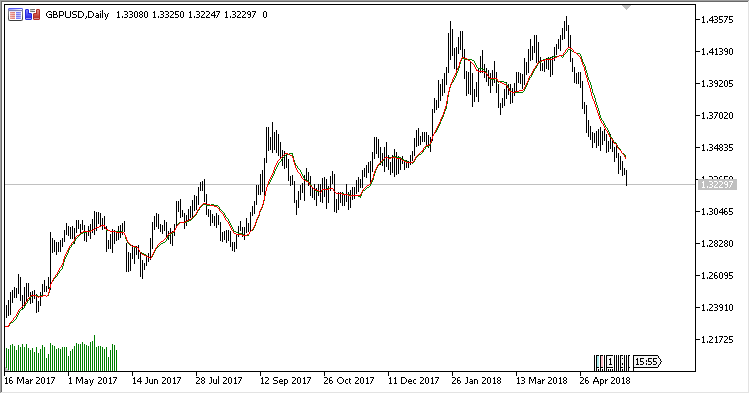Interesting script?
So post a link to it -
let others appraise it

You liked the script? Try it in the MetaTrader 5 terminal# WEVOMO - indicator for MetaTrader 5

Views:
1494
Rating:
Published:
2018.06.18 16:25
Updated:
2018.10.09 11:05

The indicator displays in the price chart two moving averages: Volume Move-Adjusted Moving Average and Weight Volume Move-Adjusted Moving Average, calculated by the formula:

```VOMOMA = (MOMA + VOMA)/2
WEVOMO = (MOMA + VOMA + WMA)/3
```

where:

```MOMA[i] = (Close[i-Period+1]*Diff[i-Period+1] + Close[i-Period+2]*Diff[i-Period+2] +... + Close[i]*Diff[i])/Sum(Diff)
VOMA[i] = (Close[i-Period+1]*Volume[i-Period+1] + Close[i-Period+2]*Volume[i-Period+2] + ... + Close[i]*Volume[i])/Sum(Volume)
WMA[i] = (Close[i-Period+1]*1 + Close[i-Period+2]*2 + ... + Close[i]*Period)/LSum
LSum = (Period+1)*Period/2
Diff[i] = Abs(Close[i] - Close[i-1])
```

The indicator has three input parameters:

• Period - calculation period;
• Show Volume Move MA - display Volume Move-Adjusted MA (VOMOMA);
• Show Weighted Volume Move MA - display Weight Volume Move-Adjusted MA (WEVOMO).Translated from Russian by MetaQuotes Software Corp.
Original code: https://www.mql5.com/ru/code/20927Wiseman_HTF

Indicator Wiseman with the timeframe selection option in its input parameters.RVRResistance

An indicator of the volume / bar price range ratio with a signal line and with the option of identifying the maximum/minimum price change resistance.SSD_With_Histogram

A slow stochastic with a histogram.Smart_Money_Pressure_Oscillator

Smart Money Pressure Oscillator